# Hill equation

(diff) ← Older revision | Latest revision (diff) | Newer revision → (diff)

An ordinary second-order differential equation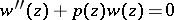with a periodic function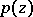; all quantities can be complex. The equation is named after G. Hill , who in studying the motion of the moon obtained the equationwith real numbers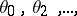where the seriesconverges.

Hill gave a method for solving this equation with the use of determinants of infinite order. This was a source for the creation of the theory of such determinants, and later for the creation by E. Fredholm of the theory of integral equations (cf. Fredholm theorems). Most important for Hill equations are the problems of the stability of solutions and the presence or absence of periodic solutions. If in the real case one introduces in the Hill equation a parameter:then, as was established by A.M. Lyapunov in , there exists an infinite sequence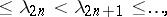such that for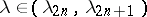the Hill equation is stable and for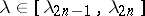it is unstable. Here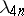and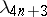are the eigen values of the periodic, and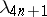and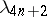the eigen values of the semi-periodic boundary value problem. The Hill equation is well studied (see ).

How to Cite This Entry:
Hill equation. Encyclopedia of Mathematics. URL: http://encyclopediaofmath.org/index.php?title=Hill_equation&oldid=18021
This article was adapted from an original article by Yu.V. Komlenko (originator), which appeared in Encyclopedia of Mathematics - ISBN 1402006098. See original article